# PSEB 9th Class Science Solutions Chapter 10 Gravitation

Punjab State Board PSEB 9th Class Science Book Solutions Chapter 10 Gravitation Textbook Exercise Questions and Answers.

## PSEB Solutions for Class 9 Science Chapter 10 Gravitation

PSEB 9th Class Science Guide Gravitation Textbook Questions and Answers

Question 1.
How does the force of gravitation between two objects change when the distance between them is reduced to half?
Let m1 and m2 be the masses of the two objects A and B respectively and ‘r’ be the distance between their centres. Therefore, according to the law of Gravitation, the force of attraction between them is given ahead: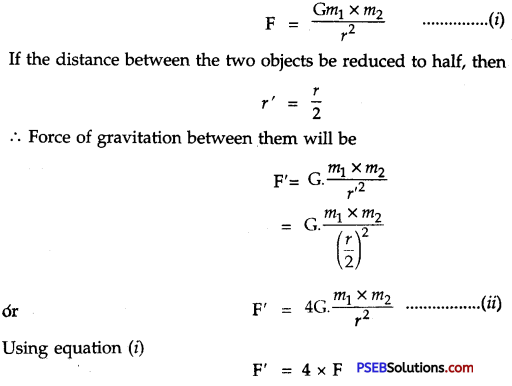Therefore, force of attraction will become four times when the distance between the two objects is reduced to half.Question 2.
Gravitational force acts on all objects in proportion to their masses. Why then, a heavy object does not fall faster than a light object?
Solution:
Suppose F is the gravitational force that acts on an object of mass’m’.
∴ F = G. $$\frac{\mathrm{M} m}{r^{2}}$$ …………….. (i)
and F = mg ………………….. (ii)
From (i) and (ii)
F = $$\frac{\mathrm{GM} m}{r^{2}}$$ = mg
It is clear that F ∝ m but acceleration due to gravity ‘g’ does not depend upon mass ‘m’. Hence all objects (light or heavy) fall with the same speed when there is no air resistance.

Question 3.
What is magnitude of gravitational force between the earth and a 1 kg object on its surface? Take mass of earth to be 6 × 1024 kg and radius of the earth is 6.4 × 106 m. G = 6.67 × 10-11 Nm2 kg-2.
Solution:
Here, mass of the object (m) = 1 kg
Mass of the earth (M) = 6 × 1024 kg
Radius of the earth (R) = 6.4 × 106 m
The magnitude of force of gravitation between object of mass 1 kg and the earth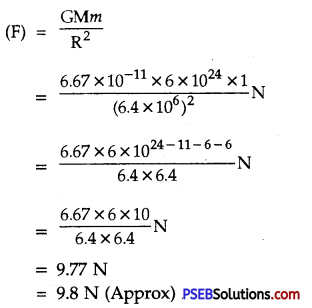Question 4.
The earth and the moon are attracted to each other by gravitational force. Does the earth attracts the moon with a force that is greater than or smaller than or the same as the force with which the moon attracts the earth? Why?
The earth attracts the moon with the same force as the force with which the moon attracts the earth. According to Newton’s third law., these two forces are equal and opposite.

Question 5.
If the moon attracts the earth, why does the earth not move towards the moon?
According to Newton’s third law, the moon also attracts earth with a force equal to that with which the earth attracts the moon. But the earth is much larger than the moon. So, the acceleration produced in the earth (a ∝ 1/m) is very less and is not noticeable.Question 6.
What happens to the force between two objects, if

1. the mass of one object is doubled?
2. the distance between the objects is doubled and tripled?
3. the masses of both objects are doubled?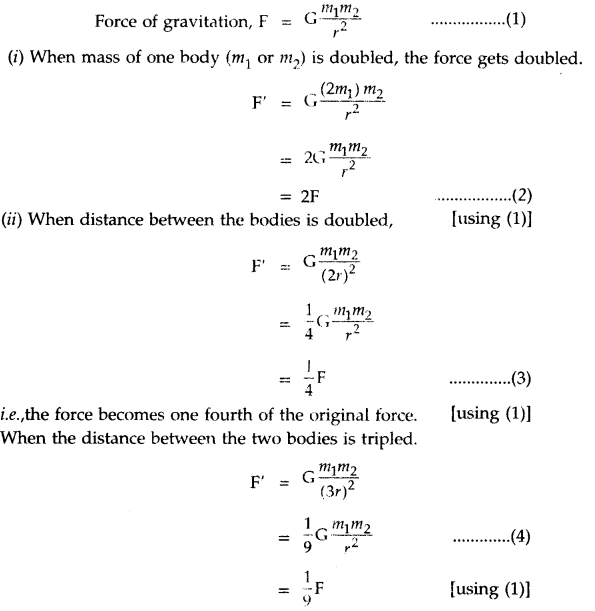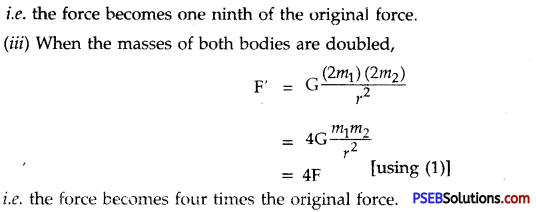i.e. the force becomes four times the original force.

Question 7.
What is the importance of universal law of gravitation?
Importance of universal law of gravitation:

1. The gravitational force between the sun and the earth makes the earth move around the sun with a uniform speed.
2. The gravitational force between the earth and the moon makes the moon move around the earth with uniform speed.
3. The high and low tides are formed in sea due to the gravitational pull exerted by the sun and the moon on the surface of water.
4. It is the gravitational pull of the earth, which holds our atmosphere in place.
5. The gravitational pull of earth keeps us and other bodies firmly on the ground.

Question 8.
What is the acceleration of free fall?
It is the acceleration produced when a body falls under the influence of the force of gravitation of the earth alone. Near the surface of the earth, its value is 9.8 m s-2.

Question 9.
What do we call the gravitational force between the earth and an object?
The gravitational force between the earth and an object is called weight of the object.Question 10.
A person ‘A’ buys few grams of gold at poles as per the instruction of one of his friends. He hands over the same when he meets him at the equator. Will the friend agree with the weight of gold bought? If not, why?
[Hint: The value of g is greater at the poles than at the equator.]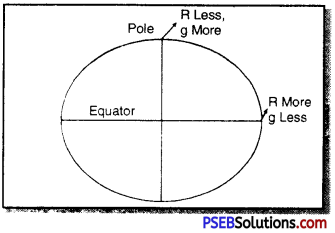The value of g at the equator is less than that at the poles. Hence, the few gm of gold at poles will measure less when taken to the equator. Therefore, the friend will not agree with the weight of the gold bought.

Question 11.
Why will a sheet of paper fall slower than one
The sheet of paper will experience a larger air resistance due to its large surface area than that of its ball form.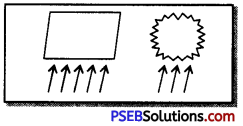Increased force of friction will reduce the forward driving force due to gravity. Hence sheet of paper falls slower than one that is crumbled into a ball.

Question 12.
Gravitational force on the surface of moon is 1/6th as strong as gravitational force on the earth. What is the weight in newton of a 10 kg object on moon and on the earth?
Solution:
Mass of the object on moon = 10 kg
Mass of the object on the earth = 10 kg
Acceleration due to gravity on the earth (g) = 9.8 m s-2
Weight of the object on the earth (W) = m × g
= 10 × 9.8
= 98 N
Now weight of the object on moon’s surface = $$\frac {1}{6}$$ × weight of the object on earth
= $$\frac {1}{6}$$ × 98N
= 16.3 N

Question 13.
A ball is thrown vertically upwards with a velocity of 49 m s-1. Calculate :
1. The maximum height to which it rises
2. The total time it takes to return to the surface of earth.
Solution:
1. Here initial velocity of the ball (u) = 49 m s-1
[At maximum height the ball comes to rest]
Final velocity of the ball (υ) = 0
Acceleration due to gravity (g) = – 9.8 m s2 [in the upward direction]
Time to reach the maximum height (t) =?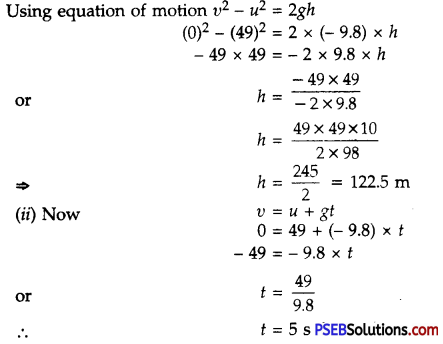∴ Total time taken to return to earth = Time for upward journey + Time for downward journey
= t + t
= 2 t
= 2 × t
= 2 × 5 s
= 10 s

Question 14.
A stone is released from the top of a tower of height 19.6 m. Calculate the final velocity just before touching the ground.
Solution:
Here, the height of the tower, (h) = 19.6 m
Initial velocity of stone, (u) = 0
Acceleration due to gravity, (g) = + 9.8 m s-1
Final velocity of the stone, (υ) = ?
Using equation of motion, υ2 – u2 = 2gh
υ2 – (0)2 = 2 × 9.8 × 19.6
υ2 = 19.6 × 19.6
υ = $$\sqrt{{19.6 × 19.6}}$$
or υ = 19.6 ms-1Question 15.
A stone is thrown vertically upward with an initial velocity of 40 m s-1. Taking g = 10 m s-2, find the maximum height reached by the stone. What is the net displacement and the total distance covered by the stone?
Solution:
Initial velocity of the stone, (w) = 40 ms-1,
Final velocity of the stone on reaching maximum height (υ) = 0 [At rest]
Acceleration due to gravity, (g) = – 10 m s2 [upward direction]
Maximum height reached, (h) = ?
We know υ2 – u2 = 2gh
(0)2 – (40)2 = 2 × (- 10) × h
– 40 × 40 = – 2 × 10 × h
∴ h = $$\frac {-40×40}{-2×10}$$
= 80 m
Since stone goes 80 m upwards and then returns to the point of throw by moving 80 m downward.
∴ Total distance travelled by stone = h + h
= 2 h
= 2 × 80 m
= 160 m
As the stone returns to the initial point of throw, therefore, net displacement is zero (0)

Question 16.
Calculate the force of gravitation between the earth and the sun, given the mass of earth = 6 × 1024 kg and of the sun = 2 × 1030 kg. Average distance between the two is 1.5 × 1011 m.
Solution:
Given, mass of the earth (m1) = 6 × 1024 kg
Mass of the sun, (m2) = 2 × 1030 kg
Average distance between the earth and the sun (d) = 1.5 × 10-11 m
G = 6.7 × 10-11 N – m2 /kg2
Force of gravitation (F) = ?
According to the universal law of gravitation,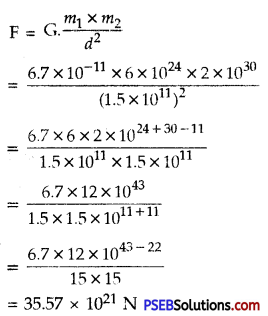Question 17.
A stone is allowed to fall from the top of the tower 100 m high and at the same time another stone is projected vertically upwards from the ground with a velocity of 25 m s-1. Calculate when and where the two stones will meet? (g = 10 ms-2)
Solution: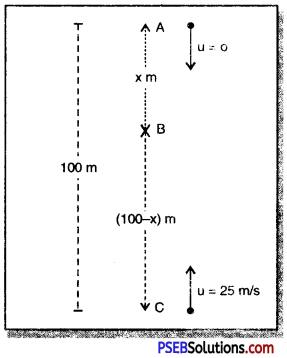Height of the tower = 100 m
Suppose a stone is allowed to fall from point A at the top of tower and another stone is projected vertically upward from point C. Let us suppose that these two stones meet at point B after ‘t’ seconds.
Distance covered by first stone (AB) = x
∴ Distance covered by second stone (CB) = (100 – x)
Downward Journey of first stone
u = 0
g = + 10 m s-2
(S) = x metres
using S = ut + $$\frac {1}{2}$$gt2
x = 0 × t + $$\frac {1}{2}$$ × 10 × t2
x = 0 + 5 × t2
⇒ t2 = $$\frac {x}{5}$$ …………..(1)
Upward journey of second stone
u = 25 ms-1
(S) = (100 – x) metres
g = – 10 m s-2
using S = ut + $$\frac {1}{2}$$gt2
(100 – x) = 25 × t + $$\frac {1}{2}$$(-10) × t2
(100 – x) = 25t – 5t2
or 5t2 = 25t – 100 + x
From (1) and (2)
$$\frac {x}{5}$$ = $$\frac {25t-100+x}{5}$$
or x = 25t – 100 + x
0 = 25t – 100
25t = 100
∴ t = $$\frac {100}{25}$$ = 4s
Now substituting the value of t = 4s in (1)
(4)2 = $$\frac {x}{5}$$
16 = $$\frac {x}{5}$$
∴ x = 16 × 5 = 80 m
i.e. the first stone will cover a distance of 80 m in the downward direction, and second stone will cover upward distance = 100 – x
= 100 – 80
= 20 mQuestion 18.
A ball thrown up vertically returns to the thrower after 6 s. Find

1. Velocity with which it was thrown up,
2. the maximum height it reached; and
3. its position after 4 s.

Solution:
Total time taken (t) = 6 s
Time taken by the ball for upward joumey= Time taken by the ball for downward journey
= $$\frac {6s}{2}$$
= 3 s
(i) Suppose the ball is thrown upwards with initial velocity u
g = – 9.8 m/s2
t = 3 s
υ = 0 [the ball stops on reaching the maximum height]
Maximum height(S) = h
using υ = u + gt
0 = u + (-9.8) × 3
0 = u – 29.4
∴ u = 29.4 ms-1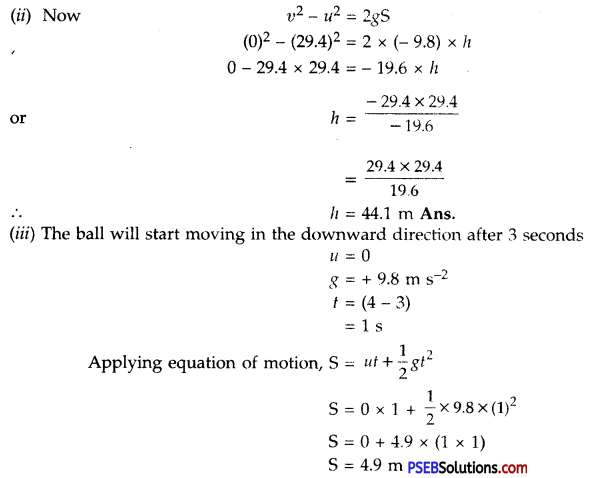∴ Height of the ball from the thrower = (44.1 – 4.9) m
= 39.2 m

Question 19.
In what direction does the buoyant force on an object immersed in a liquid act.
If an object is immersed in a liquid then the buoyant force due to displaced liquid acts on the object in vertically upward direction.

Question 20.
Why does a block of plastic immersed under water come to the surface of water?
Or
Give reason why, a block of plastic when immersed underwater comes up to the surface of water.
As density of plastic is less than the density of water. The upward thrust applied by displaced water on the plastic will be more than the weight of the plastic. So plastic block will float on water.

Question 21.
The volume of 50 g of a substance is 20 cm3. If the density of water is 1 g cm-3, will the substance float or sink?
Solution:
Here, density of water, pw = 1gm cm-3
Mass of substance, m = 50g
Volume of substance, V = 20cm3
We know, density of substance, ρ = $$\frac {m}{v}$$
= $$\frac{500 \mathrm{~g}}{350 \mathrm{~cm}^{3}}$$
= 2.5 g cm-3
As the density of the substance is greater than the density of water, the given substance will sink in water.

Question 22.
The volume of 500 g sealed packet is 350 cm3. Will the packet float or sink in water if the density of water is 1 g cm-3 ? What will be the mass of the water displaced by this packet?
Solution:
Here, mass of the packet (m) = 500 g
Volume of packet (V) = 350 cm3
∴ Density of sealed packet ρ = $$\frac {m}{v}$$
= $$\frac{500 \mathrm{~g}}{350 \mathrm{~cm}^{3}}$$
= 1.43 gcm-3
But Density of water ρw = 1 g cm-3
As density of sealed packet is more than that of water, the sealed packet will sink in water.
∴ Volume of sealed packet immersed in water = V = 350 cm3
Weight of water displaced by the packet = Vρw
= 350 × 1
= 350g.

Science Guide for Class 9 PSEB Gravitation InText Questions and Answers

Question 1.
State the universal law of gravitation.
Newton’s universal law of gravitation. This law states that every object in the universe attracts every other object with a force which is proportional to the product of their masses and inversely proportional to the square of the distance between them. This force always acts along the line joining their centres.

If m1 and m2 are the masses of two objects lying distance d apart, then force F between them is:
F = $$\frac{\mathrm{Gm}_{1} m_{2}}{d^{2}}$$
where G is a constant, called universal gravitational constant.

Question 2.
Write the formula to find the magnitude of gravitational force between the earth and an object on the surface of the earth.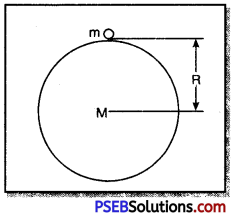Let ‘m’ be the mass of object on the earth and the mass of earth be ‘M’. If ‘R’ is the radius of the earth, then the formula for gravitational force between earth and object is:
F = $$\frac{\mathrm{Gm} M}{R^{2}}$$
Since the size of the object is very small as compared to that of the earth, therefore distance between centre of object and centre of the earth is taken to be equal to radius of the earth.Question 3.
What is meant by Free Fall?
Free Fall: An object is said to be in a state of free fall when it falls towards the earth under the influence of gravitational force between the object and the earth. There is no change in the direction of motion of the body but value of velocity keeps changing due to attraction of earth.
It falls towards earth with an acceleration of 9.8 m s-2.

Question 4.
What is meant by acceleration due to gravity?
Acceleration due to gravity: The acceleration produced in the motion of a body falling under the force of gravity is called acceleration due to gravity. It is denoted by ‘g’.

Question 5.
What is the difference between the mass of an object and its weight?
Difference between mass and weight:

 Mass Weight 1. Mass is the quantity of matter contained in a body and is the measure of its inertia. Weight of a body is the force with which a body is attracted towards the centre of the earth. 2. Mass of a body remains constant at all places. Weight of a body (W = mg) changes from place to place due to the change in the value of acceleration due to gravity ‘g’. 3. Mass is a scalar quantity. Weight is a vector quantity. 4. Mass is measured by a pan balance. Weight of a body is measured by a spring balance. 5. Mass of a body is never zero. Weight of a body is zero at the centre of the earth. 6. The unit of mass is kg. The unit of weight is newton or kg-wt.

Question 6.
Why is the weight of the object on moon -th of its weight on the earth?
We know that, Mass of earth (Me) = 100 × Mass of moon (Mm)
Since the mass and radius of moon is less than that of the earth therefore, moon exerts lesser $$\frac {1}{6}$$th force of attraction on the object. Hence the weight of the object on moon is $$\frac {1}{6}$$th of the weight of the same object on earth.

Question 7.
Why is it difficult to hold a school bag having a strap made of thin and strong string? (Imp.)
We know force per unit area is called pressure i.e. P = $$\frac {F}{A}$$. Now for the constant force, the pressure experienced is inversely proportional to area. Now, when the string is thin, it has less area of cross-section and hence, exerts greater pressure on the hand for the given weight of school bag. Thus, it becomes difficult to hold the school bag.Question 8.
What do you mean by buoyancy?
Buoyancy means upward thrust acting in a body when the body is completely or partly immersed in a fluid (i.e. liquid or a gas).

Question 9.
Why does an object float or sink when placed on the surface of water?